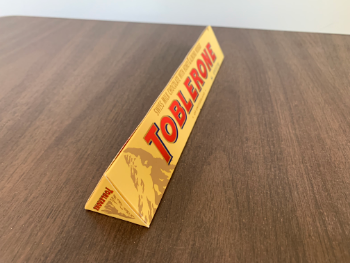# Building Surfaces with Cross Sections and Function Modeling

## Note the dimensions of the Toblerone shown below.Bases are equilateral triangles Triangle Side = 3.5 cm Prism height = 21 cm

The Toblerone candy bar shown is an example prism. By definition, what exactly makes a 3D solid a prism? Describe.

## This solid has 45-45-90 triangle cross sections parallel to the yAxis (leg lies in base). Create a solid with a base that is similar to these cross sections so that its (base) area = 50.

Which of the following solids has a volume that can be expressed as a single definite integral and not the sum of one or more definite integrals? Check all that apply.

Check all that apply